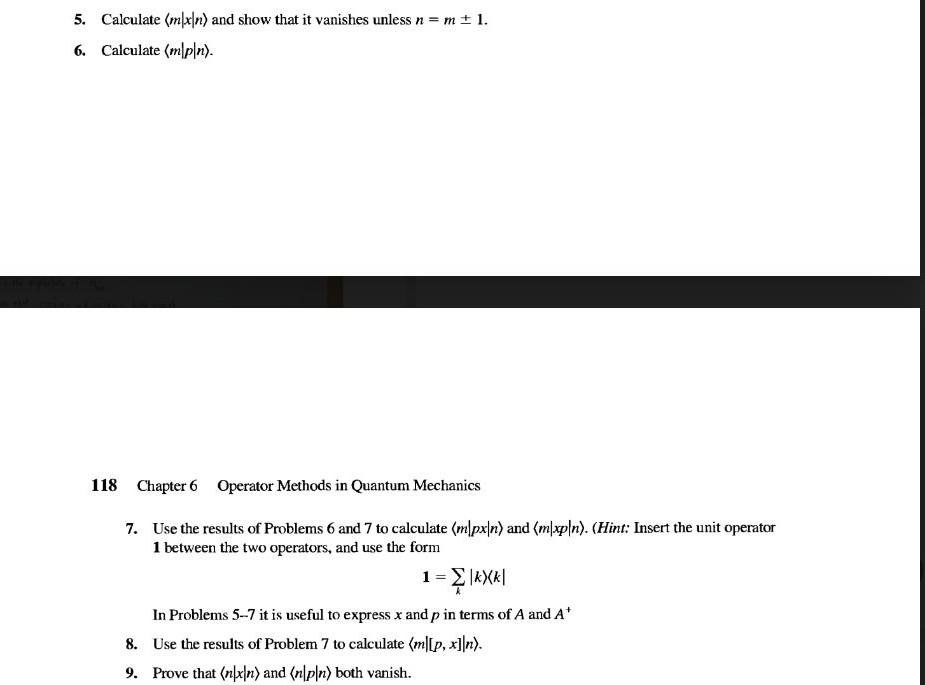# (Solved): please help me solve problem 7, 8, 9, thanks. 5. Calculate mxn and show that it van ...5. Calculate and show that it vanishes unless . 6. Calculate . 118 Chapter 6 Operator Methods in Quantum Mechanics 7. Use the results of Problems 6 and 7 to calculate and . (Hint: Insert the unit operator 1 between the two operators, and use the form In Problems 5-7 it is useful to express and in terms of and 8. Use the results of Problem 7 to calculate . 9. Prove that and both vanish.

We have an Answer from Expert

7. Using the hint and the results from Problems 5 and 6, we have:

Similarly,

We have an Answer from Expert